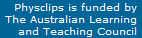Gravity, Newton's laws and the orbits of the planets

This is a supporting page to the multimedia tutorial on gravitation in Physclips. In some parts, it gives a little more detail and discussion, and it has some derivations and appendices.
 Animation showing Kepler's 3rd law

Gravity in context in physics

Gravity is a puny force. If you stand next to a tank containing many tons of water, you don't even notice its gravitational force on you. As we shall see, to produce substantial gravitational force, one of the objects has to approach planetary size. Let's compare gravity with electricity: A hydrogen atom is an electron and a proton. The electric attraction between them is larger than the gravitational attraction by a factor of 5 x 1039.

The attractive force that holds the nucleus together -- aptly called the strong force -- is stronger than the electric force over short range: if the nucleus is small enough, the strong force 'wins' and the nucleus is stable. (However, large nuclei are unstable: they divide by radioactive decay: elements with atomic number above about 90 are usually radioactive because the electrical repulsion among 90 protons tends to defeat the attraction of the strong force.) This appendix has a chart showing the known forces and some relations among them.

So why does gravity rule the universe?

• Nuclear forces only have a finite range ­ about the size of nucleus. (This finite range is determined by Heisenberg's Uncertainty Principle, using an argument that won a Nobel Prize for Hideki Yukawa.) Electromagnetism and gravity both have very large, perhaps infinite range.
• Electric charge comes in positive and negative, which cancel. The earth contains almost exactly the same number of protons and electrons, so its electrical field is small. In contrast, mass is always positive and, for gravity, every mass attracts every other mass.
So gravity runs the planets, the stars, the galaxies, galactic clusters... Let's see how.

Forces, acceleration and Newton's laws of motion

Before Galileo and Newton, Aristotle's writings dominated European thought about physics. Oversimplifying a little, it was thought that:

• things on earth fall or rise because they find their 'natural' place
• planets and other heavenly bodies move due to various, usually supernatural forces: in ancient Greek metaphor, they are pushed by the sirens and fates. There was thought to be no connection between the two: in fact, natural and supernatural were usually contrasted. Newton's laws, however, apply to both, and the first and second laws (F= ma) state that:

Only acceleration requires force

Acceleration is the rate of change in velocity. Imagine you're driving at a fixed speed: you push the accelerator, and your speed increases: this is a positive forwards acceleration. But you also need brakes to stop: a force is required to decelerate. Forces cause accelerations, and also decelerations, which are just negative accelerations.

One of Galileo's and Newton's insights was that the 'natural' condition was not zero speed but zero acceleration. If there are no forces -- no friction, no air resistance etc -- then an object at rest remains at rest, and a moving object continues moving at a constant speed in a straight line. This is Newton's first law of motion.

A larger force is required to accelerate a massive truck than to accelerate a bicycle. This is included in Newton's laws of motion, which states that the total force F applied to a mass m produces an acceleration a where

F = ma.

Force and accleration are vectors, meaning that they have direction, as well as magnitude. If the total force on a body is in the North direction, then it will accelerate North. Force is measured in newtons (abbreviation N). One newton is the force required to accelerate one kg at one metre per second per second. A newton is about the weight of a small apple.

Newton's law of motion says that a force is required to get an object moving (an acceleration in the direction of its motion), or to stop it moving (a negative acceleration in that direction). A force is also required to change the direction of its motion because, as we shall see, that is also an acceleration.

Note that mass is not the same as weight. If your mass is 70 kg, then your weight on earth is 70 kg multiplied by the earth's gravitational field, which is about 10 metres per second per second. So your weight is 700 newtons. Suppose you went to the moon, where the gravitational field is six times smaller. Your mass would still be 70 kg -- you would still be made of the same amount of material -- but your weight would be only 120 newtons. On the moon, you would fall more slowly and it would take less force to hold yourself upright, but it would still take the same force to accelerate you.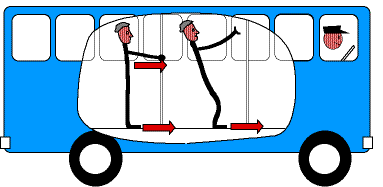A change in motion requires a force You're standing in the bus when it starts moving forwards (when it accelerates forwards). You are holding onto the grab bar. The floor of the bus pulls your feet forward, the grab bar pulls your arm and body forward - you accelerate forward with the bus. Another passenger is not holding the bar. His feet accelerate forward with the bus, but not his body. The bus accelerates forwards, he falls backwards. When the bus driver brakes, the reverse applies. The bus and the rail apply a backwards force on you, and you decelerate (ie accelerate in the negative or backwards direction). The body of other passenger, however, continues forwards while the bus slows. The bus decelerates (ie accelerates backwards), he falls forwards.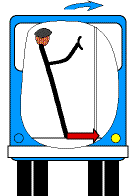Circular motion requires centripetal acceleration

What happens when the bus turns to the right, at constant speed? According to Galileo and Newton, an unrestrained passenger would tend to continue travelling in a straight line. For the passenger not holding the grab bar, his feet turn the corner with the bus, but his body keeps going forwards. The result is that he falls to the left. In this case, the bus accelerates to the right, he falls to the left.

(When you try this experiment, make sure that the person sitting on your left is simpatico.)

In circular motion, we derive an expression for the centripital acceleration: the acceleration towards the centre in circular motion. For an object moving at speed v around a circle with radius r, the acceleration is

ac = v2/r.
The period T is the time taken for one circle, so v = 2πr/T. So we can also write
ac = 4π2r/T2.
(Without maths, we can still explain in an inexact way why it has this form: the bigger v is, the larger the acceleration, because it goes from forwards at v to backwards at v twice per cycle. But the cycle repeats at a rate proportional to v/r, so it is proportional to both v and to v/r. For a real explanation, you need the maths, which is given below.)

So an object travelling in a circle at constant speed is always accelerating but, because the acceleration is towards the centre of the circle (it's called a centripetal acceleration) it doesn't add to or subtract from the speed. A force that causes a centripetal acceleration is called a centripetal force. Let's now look at some accelerations and forces.

Newton's law of gravity

In 1687, Isaac Newton wrote "I deduced that the forces which keep the planets in their orbs must be reciprocally as the squares of their distances from the centres about which they revolve; and thereby compared the force requisite to keep the Moon in her orb with the force of gravity at the surface of the Earth; and found them answer pretty nearly."

Here, \translated into modern units, is Newton's calculation of the centripital acceleration of the moon due to its circular orbit around the earth. Its direction is towards the earth. We can work out its magnitude from our equation ac = 4π2r/T2, using the distance from the earth to the moon (380,000 km) and its period of 27.3 days.I don't know whether Newton ever thought about falling apples, but if he did, he would have known that (in today's units) they fall at 9.8 ms-2. So the ratio of the accelerations is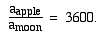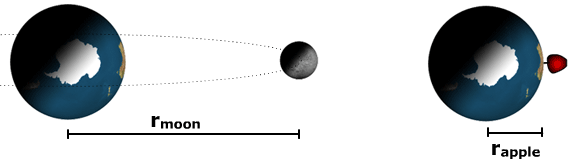Now we might expect that the effect of the earth's gravity on the moon would be less, because it is further away than the apple. For the apple, the distance from the earth's centre is the earth's radius: Re = 6370 km. So the ratio of the distances is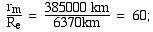so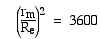Hence Newton's idea: what if the apple and the moon accelerate according to the same law? What if every body in the universe attracts every other, via an inverse square law? Note the contrast with the Aristotelean ideas mentioned above: for Newton, the same laws govern the 'heavens' as govern familiar objects. (One could see an extension of this idea as the ambit of contemporary physics: to find a simple set of laws that explain everything, from the biggest to the smallest, over the whole history of the universe.)

In modern notation, we write Newton's law thus: if two bodies with masses m1 and m2 are separated by a distance r, then the attraction between them isThere are several things to note:
• The negative sign is used to mean an attraction. If m1, m2 and the separation between them are positive (and they always are -- there are no negative masses), then the gravitational force between them is attractive. As we saw above, this is why gravity always wins on the large scale.
• The force is proportional to each of the masses. This is not surprising: experimentally we find that two apples, side by side, fall with the same acceleration as one apple, so the force on twice the mass is twice the force on one.
• There is a constant of proportionality, G, the universal constant of gravitation, which we can measure – see below.
• Gravitational forces, like all forces ever discovered, come in pairs that are equal and opposite. If m1 attracts m2 with a force F, then m2 attracts m1 with a force -F. The observation that all forces come in such pairs is Newton's third law of motion.
• The r2 in the denominator means that the attraction gets weaker in proportion as the square of the separation.

Why is gravity inverse square? is an interesting question, and one explanation is geometrical. If we picture field lines radiating uniformly from a symmetrical mass distribution, the intensity of the field (the number of field lines per unit area) is the total number of field lines (say N) divided by the area of a sphere, which is 4πr2. So the field strength is N/4πr2.

This description is rarely used for gravity. In electricity, however, the analogous relation between geometry and the strength of the field is standard and is called Gauss' Law. Magnetism is also an inverse square law, and one of Maxwell's equations is usually called Gauss' law for magnetism. If we were to use the same mathematical formulation for gravity as for electricity and magnetism, we should also have a factor 4π in the denominator. However, for gravity, the factor (1/4π) is absorbed in the constant G.

So the 1/r2 factor that it comes from the fact that we live in a universe with three dimensional*, approximately flat spatial geometry. In a flat space, the interior angles of triangles add to 180°, the area of a sphere is 4πr2 and electromagnetic and gravitational force are proportional to 1/r2. If the angles of a triangle added to less than 180°, then these forces would decrease more strongly with r and conversely.

* This argument applies only to dimensions that are large enough to be measured. Gauss' law does not rule out the existence of further dimensions, provided that the entire size of the universe in these dimensions is smaller than the smallest size we can measure. This is the escape clause available to supporters of m theory and string theory. One could argue that some nuclear physics experiments measure on the fm scale, so that the size of the universe in any putative higher dimensions must be rather smaller than this.

Inertial mass, gravitational mass and Mach's principle Why does gravity seem to depend on the (inertial) mass? This is another interesting and profound question: the same quantity, mass, that appears in Newton's equations of motion, also appears in Newton's (or Einstein's) law of gravitation. This is not due to a lack of imagination on Newton's part: he thought carefully about this coincidence.

Because the two are conceptually distinct, let's separate them. The inertial mass mi is the constant of a body that appears in Newton's combined first and second law, F = mia. It is the constant that quantifies the body's reluctance to be accelerated. The gravitational mass mg is the constant of a body that appears in Newton's law of gravitation, F = − GmgMg/r2. It quantifies the body's interaction with the gravitational field. Why are they the same? Experimentally, they are the same: Newton and many others since have conducted experiments. Or rather, they are proportional, because we could consider G to include any fixed ratio mi/mg.

What sort of experiment? Here's an example: take a pendulum consisting of a light hollow container suspended on a light string. We then fill the container with the substance to be tested. In the analysis of the pendulum, the restoring force is proportional to mg. The acceleration is inversely proportional to mi. If the two are proportional, then we find that the period of the pendulum does not depend on the mass. So, we can conduct a series of tests on different substances to see whether the period varies. For instance, if we used water or octane, we should have a relatively high ratio of protons to neutrons. If we use a heavy metal, a low ratio of protons to neutrons. So we can test whether mg/mi varies between protons and neutrons.

Another sort of experiment would be to compare the accelerations of objects in one or more gravitational fields. We give examples in this link.

One reponse is that this it is not a coincidence, and that the interactions among masses give rise to inertia. This is called Mach's Principle. Another approach is to propose that mi and mg are not merely proportional, but really are the same thing. This is a starting point for Einstein's theory of General Theory of Relativity. The proportionality of mi and mg has been and is still being tested in a number of several very sensitive experiments. We discuss it in the section on gravitation in this link.

Newton's shell theorem

Newton showed that the gravitational effect of a spherically symmetric body is the same as it would be if all its mass were located at its centre (provided that you are outside the body). Planets and stars are nearly spherically symmetric, so one can calculate their gravitational effects using separations from their centres. To prove this requires some mathematics, so we do that in this separate file.

Planetary motion

and what has been known, after a metaphor used sometimes attributed to Pythagoras, as "the music of the spheres". For two millenia or more, understanding the motion of the planets was one of the great goals of philosophers. This appendix may give some idea of the importance attached to this question.

As long ago as the fifth century BC, Leucippus and Democritus proposed a heliocentric universe, i.e. one in which the planets orbit the sun. On the mistaken assumption that the earth's motion ought to be noticeable, Hipparchus (second century BC) and Ptolemy (second century AD) proposed a universe in which the sun and planets executed complicated motions around the earth.

(Hipparchus, Ptolemy, Aristotle and many others were mistaken because they expected that one would sense velocity. In fact what we sense are forces – on our bodies, including parts of our inner ears – so our sense of motion comes from the forces required to produce the accelerations associated with the motion. As we show in the multimedia tutorial on Physclips, and also below the acceleration due to the rotation of the earth is only 0.034 m.s−2 at the equator and less at other latitudes. The force required to produce this acceleration is hundreds of times smaller than the usual forces required to support our weight. Further, it is very nearly constant: it changes its direction very slowly. So one cannot feel – nor even easily measure – the effects of the accelerations produced by the earth's rotation. For a demonstration, however, see the Foucault pendulum. Another criticism of the heliocentric model was the apparent absence of parallax in the positions of the stars. Before the development of good telescopes, this was difficult to observe, and Aristarchus proposed that they were infinitely distant.)

The Danish astronomer Tycho Brahe (1546-1601) made very many, very careful, naked eye observations of the positions of the planets. He was joined by Johannes Kepler, a tireless calculator. After a long time trying to fit circular orbits and even musical harmonies to the data, Kepler eventually discovered that the data were all well fitted by the following empirical laws.

Kepler's laws

• 1. All planets move in elliptical orbits, with the sun at one focus.
The elliptical orbits of the planets are, however, not very far from being circles. (Mars was the most eccentric orbit that Kepler studied. The orbit of Pluto – unknown in Kepler's time – is much more eccentric.) The mass of the sun is so much greater than that of the planets that the sun is very nearly the centre of mass of the solar system. (We return to this approximation below.)
• 2. A line joining the planet to the sun sweeps out equal areas in equal time.
i.e. Planets move more slowly at apogee (distant), more rapidly at perigee (close).
• 3. The square of the orbital period (i.e. the planet's 'year') is proportional to the cube of the semi-major axis of its orbit.
Slow orbits for distant planets, fast for close.
• Why is it so? What underlying reasons are there for Kepler's laws? It is difficult for a modern person to have a feeling for the magnitude of this puzzle – hence this appendix. For two millenia, this was the riddle of riddles. So Newton's demonstration that the three laws follow simply from his laws of mechanics was a major triumph and secured his fame. Proving the first law requires a reasonable amount of geometry, so we omit it here. In the multimedia tutorial on Physclips we derive the other two. To revise briefly here:

For circular motion, with the sun at the centre (see below), the centripital acceleration is, as before

ac = rω2 = 4π2r/T2,
where r is the radius, ω the angular velocity and T the period. Now add a force whose magnitude is GmM/r2 and set F = ma:
GmM/r2 = Fgravity = mac = m4π2r/T2,   so:

T2 = (4π2/MG)r3      (K2)

For circular orbits at least, we have derived Kepler's third law in a few simple equations. The same force that makes the apple fall rules the heavens. The second law, which is animated below, follows just as simply from conservation of angular momentum. The proof, which is given in the multimedia section is repeated here:

 For small angle δθ, the area subtended by δθ is that of a triangle with long side r: δA = ½r.(rδθ). The gravitational force acting towards the sun exerts no torque on the planet, so the angular momentum L about the focus of the orbit is conserved. If the momentum of the planet is p = mv, |L|  =  |r X p|  =  |r X mv|   =  mrvtangential (You can revise vectors.) In terms of the angular velocity ω, this gives |L|  =  mr.rω  =  mr2(δθ/δt)  =  m(r2δθ)/δt and, using the expression for δA |L|  =  2mδA/δt So, because |L| is constant, then in any given time δt, δA is constant.

Satellite orbits

In the multimedia section, we use the equation derived above, T2 = (4π2/MG)r3, to derive the period of orbits for earth's satellites, using G = 6.67 x 10-11 Nm2kg-2 and Me = 5.97 x 1024 kg. Many satellites, including the international space station, are in low earth orbit, meaning an orbit with a radius a little greater than that of the earth: say 6,500 km. Substitution in (K2) gives a period of about 90 minutes. (So, in Shakespeare's A Midsummer Night's Dream, Puck seems to be out by a factor of two.)

Newton gave this nice idea to help explain orbits. First, consider a projectile (Newton imagined a cannonball) fired horizontally from the top of a mountain, over a distance small enough that the earth's surface can be considered flat. No matter what its horizontal speed, its vertical acceleration is g downwards.

If we fire it very fast, however, we can no longer neglect the curvature of the earth. At a particular value of the horizontal velocity (about 28,000 k.p.h.), the curvature of the path of the cannonball will match the curvature of the surface of the earth. The cannonball still accelerates downwards at g, but g is now the centripital acceleration of its circular motion, as shown below.

 Another very important orbit is that of geosynchronous satellites, whose period of 23.9 hours keeps them above the same point on the earth's equator. Again, using equation T2 = (4π2/MG)r3 and G = 6.67 x 10-11 Nm2kg-2 and Me = 5.97 x 1024 kg, we get an orbital radius of 42,000 km. In the animation, the size of the earth and the orbit (but not the satellite) are to scale. This orbit is very useful for communications satellites: if you point your dish towards the satellite, you don't have to move it. (We note in passing that an object at rest on the earth's surface already has a speed of ωrecos (latitude), due to the rotation of the earth. This is over 1,000 k.p.h for tropical latitudes, which provides an incentive to locate space launch centres at low latitudes. This is probably one reason why NASA launches from Florida.)

Orbits and the centre of mass

It is a common simplification to say that 'the moon's orbit is a circle about the earth': the gravitational attraction of the moon towards the earth provides the centripetal force for the moon's orbit. From Newton's third law, of course, the moon attracts the earth with a force of equal magnitude, which accelerates the earth. So the earth and the moon each trace (almost perfectly) circular orbits about their common centre of mass. The sketched below illustrates this, but is not to scale.Using subscripts m and e for the moon and earth, we can write

Magnitude of force on the moon due to earth:     Fg =   mmam  =  mmω2rm     and
Magnitude of force on the earth due to moon:     Fg =   meae  =  meω2re.
Setting these two expressions equal gives

rm/re  =  me/mm  =  (5.98 x 1024 kg)/(7.36 x 1022 kg)  =  81.3        (i)

The distance between the centres of the earth and the moon gives a second equation:

re + r m   =  3.85 x 108 m             (ii)

Solving these gives rm   =  3.80 x 108 m    and    re   = 4.7 x 106 m

So the centre of both orbits, which is also the centre of mass, is 4700 km from the centre of the earth: it lies inside the earth. (In contrast, the analogous calculation shows that the centre of mass of the sun-Jupiter system lies outside of the sun.)

A puzzle

An interesting paradox: why doesn't the moon fall into the sun? Calculate the point on a line between earth and sun at which the gravitational forces exerted on a mass m by the earth (Fe) and the sun (Fs) are equal and opposite.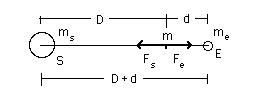Doing the calculation, we find that the point of equal attraction lies 260,000 km from the earth. But the moon is 380,000 km from the earth! At new moon, the point of equal attraction lies nearly on a line between the earth and the sun (during a solar eclipse, it lies exactly on this line). So, at new moon, will the moon fall into the sun? The problem and the calculation are described here. But I'm not giving answers: it's for you to solve!

Gravitational potential energy

When you lift something up, you expend energy -- you do work on it. This energy is stored, in the sense that you can get it back again. It is called gravitational potential energy. For instance, during periods of low electricity demand, energy authorities in Australia use electrical energy from coal-fired power stations to pump water up the Snowy Mountains into high dams. In periods of high demand, they can then let it flow back downhill to get back the gravitational potential energy of that water via the hydroelectric generators.

The gravitational potential energy U of two objects, masses M and m, at separation r, is obtained from the definition of potential energy, −Ug = ∫ Fg.ds. (Revise potential energy.) Because gravity acts in the direction of −r,  we can write Fg.ds as −Fgdr. Substituting from Newton's equation for Fg and integrating:Again, we have a constant of integration to define, which means a zero of potential energy to choose. An astronomer would tell you that there is nothing special about the surface of the earth, so the zero of potential energy is taken by astronomical convention at infinite separation, so set U = 0 at ri = ∞, which givesA consequence of this general or astronomical convention is thatgravitational potential energy is always negative at finite separation. This does not affect the main point that work must be done to lift something: gravitational potential energy increases with separation. (If M is the earth and m a small object, we speak of the U of m in the field of M. However U is a property of the pair of objects and we could equally talk of the potential energy of M in the field of m.)The graph above shows two different expressions for gravitational potential energy of a mass m near the earth. For r > re, we use U = −GMem/r. Very close to the earth (in other words when (r − re) << re), we can use the approximation GMe/re ≅ g and the binomial expansion to write:

U'  ≡  U(r) − U(re)  ≅   (GMem/r)(r − re)  ≅  mgh.

The limits to Newtonian gravity

So far, we have only mentioned Newton's theory of gravitation. Although it is an excellent theory, it does not agree with experiment if one investigates extremely large fields, or moderately large fields with very high precision. In other words, it is wrong. However, it is such an excellent approximation that Newtonian gravity is what we use to calculate in almost all circumstances, while recognising that it is just a very convenient approximation to more exact theories.

To be accepted, any new theory of gravity must give virtually the same answer as Newton's in all of the many cases where Newton's theory works, but must also give the right answer for the cases where we know that Newton's theory fails, such as the slight deflection of starlight passing very close to the sun (observable during a solar eclipse) and certain aspects of the orbit of Mercury that cannot be accounted for by considering the effects of the other planets.

Several other theories do this, of which one of the simplest and by far the most widely used is Einstein's General Theory of Relativity. Philosophically, General Relativity is very different from Newtonian gravity, in that it doesn't use forces. Rather, the effect of large masses is to curve space, and the curvature of space determines the motion of objects. It is often summarised thus: "matter tells space how to curve, and space tells matter how to move".

So, if there are several competing theories that all do better than Newton's, what theory of gravitation should we use? The mission of Gravity Probe B was to conduct experiments to distinguish among some current theories of gravitation, but it had limited results.

However, both Einstein's and Newton's theories of gravity have a problem when they encounter quantum mechanics, and that problem involves the very nature of space and time. We explain this further on Gravity, relativity and quantum mechanics.

Fortunately, the scale of the problem is that of the Planck length, which is 1.6 x 10-35 metres. This is immeasurably small, and it is perhaps not too surprising that our ideas about space would need to be revised on this scale.

(Yes, small: the Planck length is 0.000000000000000000000000000000000016 metres. Let's compare it with the size of an atom, which is already about 100,000 times smaller than anything you can see with your unaided eye. Suppose that you measured the diameter of an atom in Planck lengths, and that you counted off one Planck length each second. To measure the atomic diameter in Planck lengths would take you 10,000,000 times the current age of the universe.)

The limits to Newtonian physics

While we're on the subject, let's be quantitative about these limits, and let's look at Newtonian mechanics from other perspectives.
• Newtonian gravity is accurate if the gravitational potential energy Ugravis much less than the proper energy mc2. At the earth's surface, Ugrav/mc2 ≅ 7 x 10−10. So, unless you are looking for very high precision, Newtonian gravity is an excellent approximation. However, time is often measured very precisely, and such precision is used in navigation systems. So general relativistic corrections cannot be neglected in such applications.
• Newtonian mechanics is accurate if relative velocities are small compared with that of light. For a jet airliner, v/c < 10−6. Macroscopic objects travel much more slowly than c, but atomic particles and especially electrons are regularly accelerated to high speeds. Again, timing and navigation systems sometimes require the precision that obliges relativistic calculations.
• Newtonian mechanics is also limited by scale. It is accurate if the product of momentum and a typical length is much greater than Planck's constant, h. For a small molecule at room temperature, (momentum*size)/h ~ 10. For a molecule in a gas, the typical size of the container is large compared to the molecule, so classical mechanics is fine, unless the temperature (and therefore the molecular speeds) is very low. On the molecular scale, however, Quantum Mechanics begins to be important. For low mass objects, such as electrons, and especially at lower temperatures, momentum*size may be much less than one, and quantum effects may dominate.

Why g is not parallel to Fg

 To an excellent approximation, the gravitational force on a mass m at the earth's surface is Fg ≅ mg. An excellent approximation, but not exact (except at the poles), because of the earth's rotation: the earth's surface is not an inertial frame. A point on the earth's surface is accelerating with centripital acceleration whose magnitude is acentrip  =  rω2  =  re(cos θ) ω2 where re(cos θ) is the radius of the earth, ω is its angular velocity about its axis and θ is the latitude. When we measure the weight mg of a mass m, we typically do this by putting it on a balance and measuring the normal force N required to keep it stationary with respect to the earth – i.e. to keep it accelerating with acentrip. So macentrip  =  N + Fg    or      −N  =  Fg − macentrip. Now re(cos θ) ω2 is 0.034 m.s−2 at the equator, less at other latitudes and zero at the poles. Usually, we then make the approximation that re(cos θ) ω2 << g = 9.8 m.s−2, so N ≅ Fg.The diagram above may seem odd, because the vertical of the string is not at right angles to the horizontal. That's because, in that diagram, we exaggerated the angles of the forces, but didn't consider the effects of rotation on the shape of the earth. So, let's hang the mass on a string and leave it at rest, with respect to the earth. As shown, the tension in the string will be almost the same as mg, but not quite. The string hangs vertically, by definition of vertical. However, this direction is not quite parallel to Fg and it does not point exactly at the centre of the earth. A similar argument determines the shape of the earth. The surface of a bowl of water is horizontal, by definition. Here, the normal force and the gravitational force acting on a mass at the surface of the water must also add to give acentrip,, otherwise the water would flow out of the bowl. So the surface of the water – horizontal – is at right angles to the string in our example.

The earth's shape is determined by the the forces on it and, on a large scale, it is very close to that of a drop of spinning fluid so, the earth's (large scale average) surface is also, roughly, at right angles to the string. In the animation below, the rate of rotation is exaggerated so that the difference in angle and the tiny eccentricity of the earth's shape – both very tiny in practice – can be seen.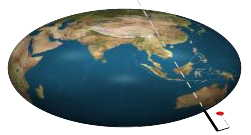What happens if you spin so fast that the string points away from the axis of the earth? The next animation shows this unhappy (but unlikely) result.

Appendix: Film clips illustrating Newton's second law

The film clips below use a spring to accelerate a bowling ball. You can tell whether the spring applies a force from whether it is stretched or not, as in these photos. Use the step frame button on the film clips.Appendix: Centripetal accleration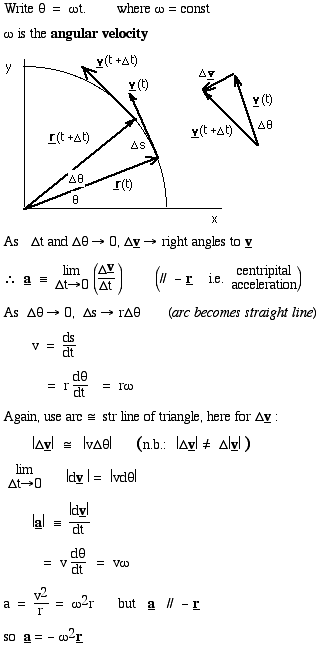See also the section on circular motion, in which this derivation is animated.

Appendix: Faust and the music of the spheres

Space travel has made us blasé about motion in the skies. This may give us some flavour of the Mediaeval view: Christopher Marlowe (a contemporary and rival of Shakespeare) created Doctor Faustus (perhaps based on the historical character Paracelsus) -- a philosopher/scientist who sells his soul for knowledge. Here is an extract from the play, in Act VI:

Faustus:    I am resolv'd: Faustus shall ne'er repent.
Come, Mephistophiles, let us dispute again,
And argue of divine astrology.
Tell me, are there many heavens above the moon?
Are all celestial bodies but one globe,
As is the substance of this centric earth?
Mephistopheles:      As are the elements, such are the spheres
Mutually folded in each other's orb,
And, Faustus,
All jointly move upon one axletree
Whose terminine is termed the world's wide pole;
Nor are the names of Saturn, Mars, or Jupiter
Feign'd but are erring stars.
Faustus:    But tell me, have they all one motion, both situ et tempore?
Mephistopheles:    All jointly move from East to West in twenty-four hours upon the poles of the world; but differ in their motion upon the poles of the zodiac.
Faustus:    Tush!
These slender trifles Wagner can decide;
Hath Mephistophiles no greater skill?
Who knows not the double motion of the planets?
The first is finish'd in a natural day;
The second thus: as Saturn in thirty years; Jupiter in twelve; Mars in four; the Sun, Venus, and Mercury in a year; the moon in twenty-eight days. Tush, these are freshmen's suppositions. But tell me, hath every sphere a dominion or intelligentia?
Mephistopheles:      Ay.
Faustus:    How many heavens, or spheres, are there?
Mephistopheles:      Nine: the seven planets, the firmament, and the empyreal heaven.
Faustus:    Well, resolve me in this question: Why have we not conjunctions, oppositions, aspects, eclipses, all at one time, but in some years we have more, in some less?
Mephistopheles:      Per inæqualem motum respectu totius*.          *On account of their unequal motion in relation to the whole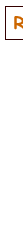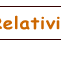This work is licensed under a Creative Commons License.